##### The Japanese Bonsai specialist
Direct order Contact Help / Services Newsletter# Set of 3 akadama bonsai soil various grain sizes

› Special offer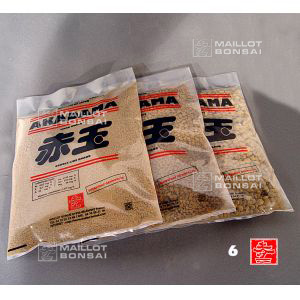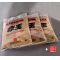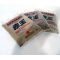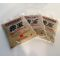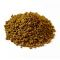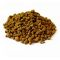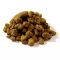ref. : 10

19,00

voluminous/heavy item extra shipping of 4,00

Available quantity : 8Order

###### Description

1 sachet super fine grain bonsai soil for small pots. No need to sieve. Grain size Ø + -1/3mm.

1 sachet medium-sized grain bonsai soil. Grain size Ø + - 2/3/4 mm.

1 sachet large-sized grain bonsai soil. Grain size Ø + -3/5 mm.

3 sachets + - 2 litres each.SUPERIOR QUALITY, DRIED FOR MORE THAN 2 YEARS. Weight according to the rate of moisture of 8.15 kilograms.

Origin: natural volcanic earth from the mountainous regions of Japan.

Benefits: soil drainage and root ventilation for the healthy growth of your bonsai.

How to use: use alone or add gravel and large-grained sand but not compost. This earth is not very nourishing and a Biogold fertiliser should be used regularly.

Species: for cultivating all coniferous and deciduous species (except rhododendrons and azaleas, for which you'll need Kanuma earth).

#grain 8.5 #bonsai 6.6 #soil 6.4 #sachet 3.6 #earth 3.5 #size 3.4 #akadama 2.7 #various 2.7 #species 2.7 #special 2.7

Formule
(( ROUND((CHAR_LENGTH(b.article_nom)-CHAR_LENGTH(REPLACE(b.article_nom, 'grain', '')))/LENGTH('grain')) + ROUND((CHAR_LENGTH(b.article_description)-CHAR_LENGTH(REPLACE(b.article_description, 'grain', '')))/LENGTH('grain')) ) * 8.5) + (( ROUND((CHAR_LENGTH(b.article_nom)-CHAR_LENGTH(REPLACE(b.article_nom, 'bonsai', '')))/LENGTH('bonsai')) + ROUND((CHAR_LENGTH(b.article_description)-CHAR_LENGTH(REPLACE(b.article_description, 'bonsai', '')))/LENGTH('bonsai')) ) * 6.6) + (( ROUND((CHAR_LENGTH(b.article_nom)-CHAR_LENGTH(REPLACE(b.article_nom, 'soil', '')))/LENGTH('soil')) + ROUND((CHAR_LENGTH(b.article_description)-CHAR_LENGTH(REPLACE(b.article_description, 'soil', '')))/LENGTH('soil')) ) * 6.4) + (( ROUND((CHAR_LENGTH(b.article_nom)-CHAR_LENGTH(REPLACE(b.article_nom, 'sachet', '')))/LENGTH('sachet')) + ROUND((CHAR_LENGTH(b.article_description)-CHAR_LENGTH(REPLACE(b.article_description, 'sachet', '')))/LENGTH('sachet')) ) * 3.6) + (( ROUND((CHAR_LENGTH(b.article_nom)-CHAR_LENGTH(REPLACE(b.article_nom, 'earth', '')))/LENGTH('earth')) + ROUND((CHAR_LENGTH(b.article_description)-CHAR_LENGTH(REPLACE(b.article_description, 'earth', '')))/LENGTH('earth')) ) * 3.5) + (( ROUND((CHAR_LENGTH(b.article_nom)-CHAR_LENGTH(REPLACE(b.article_nom, 'size', '')))/LENGTH('size')) + ROUND((CHAR_LENGTH(b.article_description)-CHAR_LENGTH(REPLACE(b.article_description, 'size', '')))/LENGTH('size')) ) * 3.4) + (( ROUND((CHAR_LENGTH(b.article_nom)-CHAR_LENGTH(REPLACE(b.article_nom, 'various', '')))/LENGTH('various')) + ROUND((CHAR_LENGTH(b.article_description)-CHAR_LENGTH(REPLACE(b.article_description, 'various', '')))/LENGTH('various')) ) * 2.7) + (( ROUND((CHAR_LENGTH(b.article_nom)-CHAR_LENGTH(REPLACE(b.article_nom, 'species', '')))/LENGTH('species')) + ROUND((CHAR_LENGTH(b.article_description)-CHAR_LENGTH(REPLACE(b.article_description, 'species', '')))/LENGTH('species')) ) * 2.7) + (( ROUND((CHAR_LENGTH(b.article_nom)-CHAR_LENGTH(REPLACE(b.article_nom, 'akadama', '')))/LENGTH('akadama')) + ROUND((CHAR_LENGTH(b.article_description)-CHAR_LENGTH(REPLACE(b.article_description, 'akadama', '')))/LENGTH('akadama')) ) * 2.7) + (( ROUND((CHAR_LENGTH(b.article_nom)-CHAR_LENGTH(REPLACE(b.article_nom, 'large', '')))/LENGTH('large')) + ROUND((CHAR_LENGTH(b.article_description)-CHAR_LENGTH(REPLACE(b.article_description, 'large', '')))/LENGTH('large')) ) * 2.5)

## Secure payment## Delivery

Our logistic partners :04 74 55 23 48
Pépinière MAILLOT-BONSAÏ
Le Bois Frazy
01990 RELEVANT - FRANCE
on appointment